Courses

# Test: Alcohols, Phenols and Ethers- 2

## 32 Questions MCQ Test Chemistry for JEE | Test: Alcohols, Phenols and Ethers- 2

Description
This mock test of Test: Alcohols, Phenols and Ethers- 2 for Class 12 helps you for every Class 12 entrance exam. This contains 32 Multiple Choice Questions for Class 12 Test: Alcohols, Phenols and Ethers- 2 (mcq) to study with solutions a complete question bank. The solved questions answers in this Test: Alcohols, Phenols and Ethers- 2 quiz give you a good mix of easy questions and tough questions. Class 12 students definitely take this Test: Alcohols, Phenols and Ethers- 2 exercise for a better result in the exam. You can find other Test: Alcohols, Phenols and Ethers- 2 extra questions, long questions & short questions for Class 12 on EduRev as well by searching above.
QUESTION: 1

###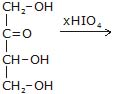x is moles of HIO4 consumed

Solution: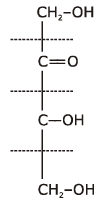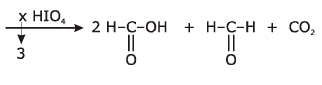QUESTION: 2

###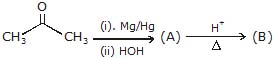Product (B) is -

Solution: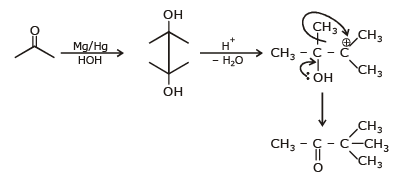QUESTION: 3

###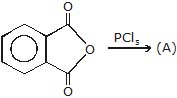; Product (A) is -

Solution: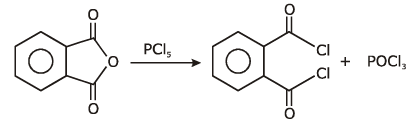QUESTION: 4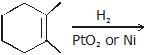Product of above reaction is obtained by

Solution: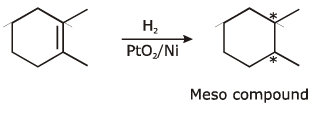QUESTION: 5

The structure of the product formed in the reaction given below is -Solution: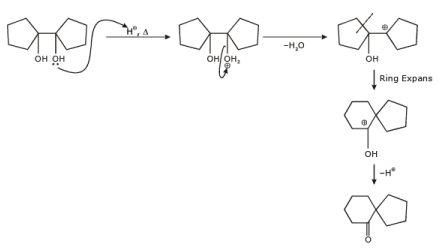QUESTION: 6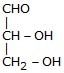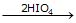, Products obtained in the above reaction are -

Solution: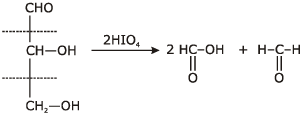QUESTION: 7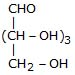+ 4HIO4 ¾→, Products obtained are

Aldo pentose

Solution: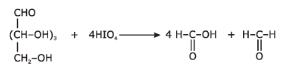QUESTION: 8

Which of the following compound gives 2HCHO, CO2, 2HCO2H when oxidisized by periodic acid

Solution: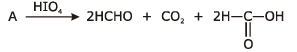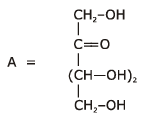QUESTION: 9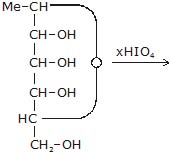,

What is the maximum value of (x) ?

Solution: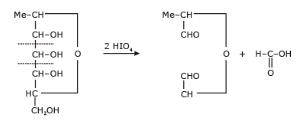QUESTION: 10

An ether is more volatile than an alcohol having the same molecular formula. This is due to

Solution:

The correct answer is Option D.
An ether is more volatile than an alcohol having the same molecular formula. This is due to intermolecular hydrogen bonding in alcohols. In alcohols, H atoms are attached to electronegative O atoms. Due to this, H atom of −OH group of one alcohol molecule forms a hydrogen bond with the O atom of −OH group of the second alcohol molecule. This results in the intermolecular association. It increases the boiling point and decreases the volatility of alcohol. Such intermolecular hydrogen bonding is not possible in others as in ethers, H atoms are not attached to an electronegative O atom.

QUESTION: 11

In the given reaction :

CH3_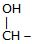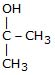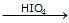(a) + (b)

(a) and (b) respectively be -

Solution: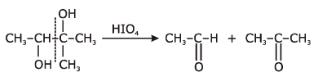QUESTION: 12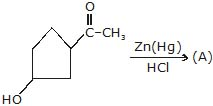;

Identify the A.

Solution: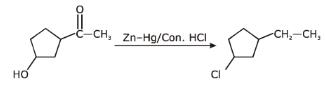QUESTION: 13

Ph–CH2–Cl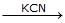(A)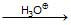(B)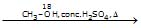(C)

product (C) is -

Solution: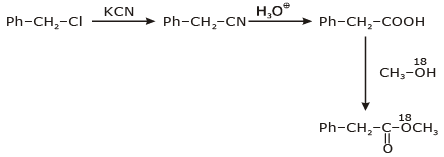QUESTION: 14

Ph–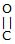–CH2–O–Et(A)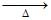(B)

Product (B) is -

Solution: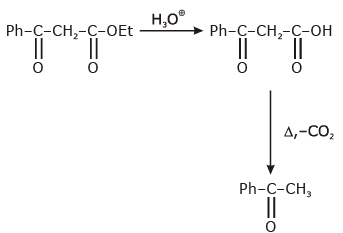QUESTION: 15

R––O–H+R1–OH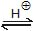R––O–R,

In above esterification reaction rate of reactin is maximum, when R1–OH is -

Solution: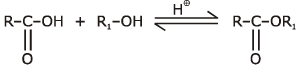Order of alcohal towards esterification
CH3–OH > 1°alcohal > 2°alcohal > 3°alchoal.

QUESTION: 16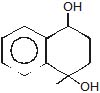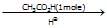(A)

product (A) is -

Solution: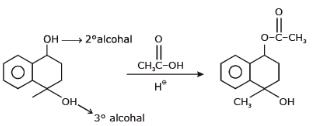QUESTION: 17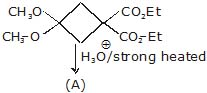;

Product (A) is -

Solution: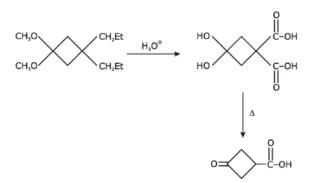QUESTION: 18

R1–OH + R – OH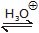R1–O–R–+H2O

In above esterification reaction rate of reaction maximum when -

Solution: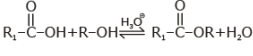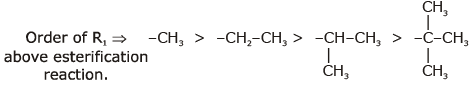QUESTION: 19

Ph–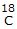–CHO18 is present in -

Solution: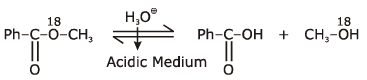QUESTION: 20

Ph––O–CH3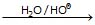Above reaction is known as -

Solution: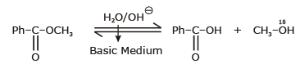Hydrolysis of ester in basic Medium is known as saponification.

QUESTION: 21

CH3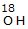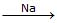(A) + B (gas)
CH3__O_CH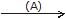(C)

Product (C) of above reaction is -

Solution: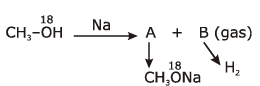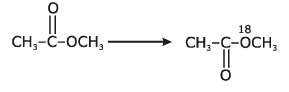QUESTION: 22

CH3–CH2–O–Et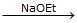(A)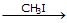(B)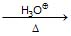(C)

Product (C) is -

Solution: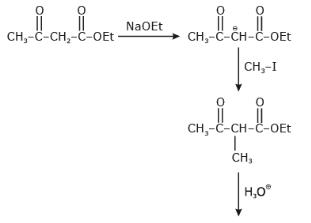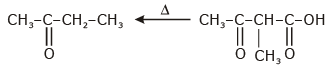QUESTION: 23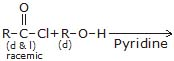Product of above reaction is -

Solution: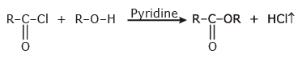HCl is formed in above reaction and react with pyridine and gives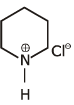QUESTION: 24

Ph––O–H+CH3–O18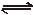(X) + H2O

Solution: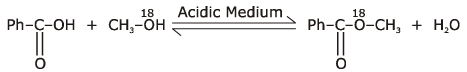This is a esterfication reaction.

QUESTION: 25

Which of the following is the least soluble in water?

Solution:

As the size of the alkyl group becomes larger, it prevents the formation of hydrogen bonds with water molecules and hence the solubility goes on decreasing with the increase in the size or mass of the compound.

QUESTION: 26

Which of the following compound reduces by DIBAL-H ?

Solution:

The correct answer is option D
At ordinary temperatures, DIBAL-H reduces a variety of carbonyl compounds, like aldehydes, ketones, carboxylic acids and esters, to corresponding alcohols.  These reductions are chemoselective as well as stereospecific.

QUESTION: 27

Which of the following compound reduces by NaBH4 ?

Solution:

NaBH4 are weak reducing agent so only acid chloride are reduced in alcohal.

QUESTION: 28

Which of the following compounds not reacts with NaOH ?

Solution: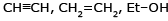are not react with NaOH.

QUESTION: 29

EtOH+CH3––O–CH3 ¾→

In above reaction molecular weight of alcohol increases by

Solution: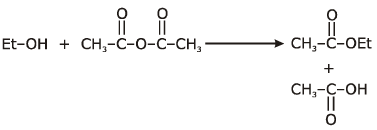in above reaction molecular weight of alcohal increase by C2H2O = 42.

QUESTION: 30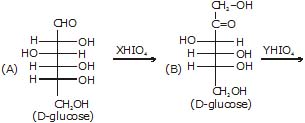x & y are moles of HIO4 consumed is above reaction.

(i) Value of x inabove reaction is -

Solution: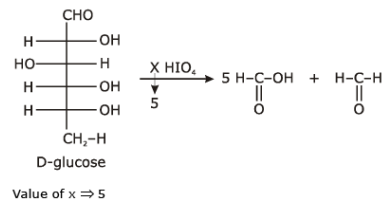QUESTION: 31x & y are moles of HIO4 consumed is above reaction.

Sum of x + y is -

Solution: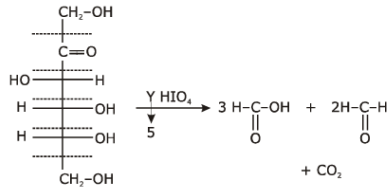QUESTION: 32x & y are moles of HIO4 consumed is above reaction.

Mole of HCHO formed in (A) is -

Solution:

Total no. of HCHO in A = 1